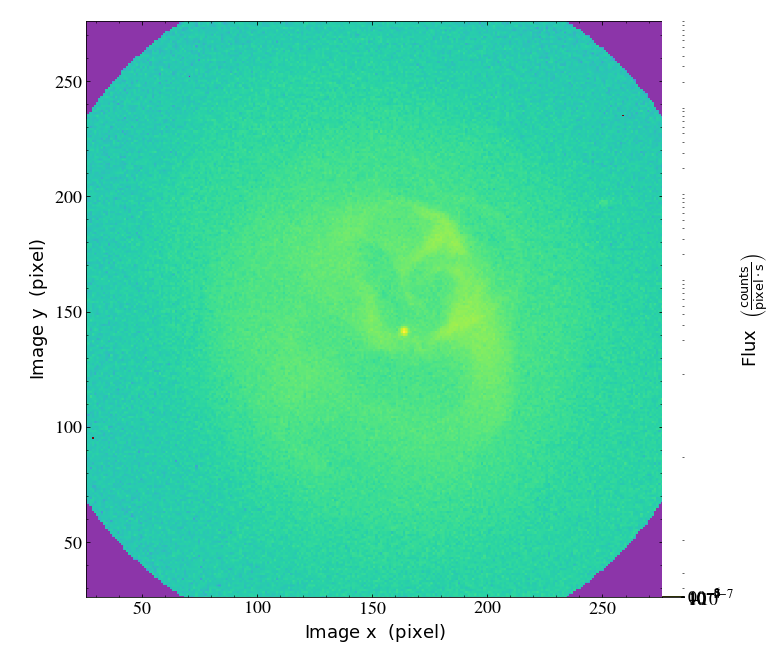# FITS X-ray Images in yt¶

Notebook
In :
%matplotlib inline
import yt
import numpy as np


This notebook shows how to use yt to make plots and examine FITS X-ray images and events files.

## Sloshing, Shocks, and Bubbles in Abell 2052¶

This example uses data provided by Scott Randall, presented originally in Blanton, E.L., Randall, S.W., Clarke, T.E., et al. 2011, ApJ, 737, 99. They consist of two files, a "flux map" in counts/s/pixel between 0.3 and 2 keV, and a spectroscopic temperature map in keV.

In :
ds = yt.load("xray_fits/A2052_merged_0.3-2_match-core_tmap_bgecorr.fits",
auxiliary_files=["xray_fits/A2052_core_tmap_b1_m2000_.fits"])


Since the flux and projected temperature images are in two different files, we had to use one of them (in this case the "flux" file) as a master file, and pass in the "temperature" file with the auxiliary_files keyword to load.

Next, let's derive some new fields for the number of counts, the "pseudo-pressure", and the "pseudo-entropy":

In :
def _counts(field, data):
exposure_time = data.get_field_parameter("exposure_time")
return data["fits", "flux"]*data["fits", "pixel"]*exposure_time

def _pp(field, data):
return np.sqrt(data["gas", "counts"])*data["fits", "projected_temperature"]

def _pe(field, data):
return data["fits", "projected_temperature"]*data["gas", "counts"]**(-1./3.)

/tmp/yt/venv/lib/python3.8/site-packages/unyt/array.py:1778: RuntimeWarning: divide by zero encountered in power
out_arr = func(


Here, we're deriving a "counts" field from the "flux" field by passing it a field_parameter for the exposure time of the time and multiplying by the pixel scale. Second, we use the fact that the surface brightness is strongly dependent on density ($S_X \propto \rho^2$) to use the counts in each pixel as a "stand-in". Next, we'll grab the exposure time from the primary FITS header of the flux file and create a YTQuantity from it, to be used as a field_parameter:

In :
exposure_time = ds.quan(ds.primary_header["exposure"], "s")


Now, we can make the SlicePlot object of the fields we want, passing in the exposure_time as a field_parameter. We'll also set the width of the image to 250 pixels.

In :
slc = yt.SlicePlot(ds, "z",
[("fits", "flux"), ("fits", "projected_temperature"), ("gas", "pseudo_pressure"), ("gas", "pseudo_entropy")],
origin="native", field_parameters={"exposure_time":exposure_time})
slc.set_log(("fits", "flux"),True)
slc.set_log(("gas", "pseudo_pressure"),False)
slc.set_log(("gas", "pseudo_entropy"),False)
slc.set_width(250.)
slc.show()

/tmp/yt/venv/lib/python3.8/site-packages/unyt/array.py:1778: RuntimeWarning: invalid value encountered in power
out_arr = func(
/tmp/yt/venv/lib/python3.8/site-packages/unyt/array.py:1778: RuntimeWarning: invalid value encountered in multiply
out_arr = func(
/tmp/yt/venv/lib/python3.8/site-packages/unyt/array.py:1674: RuntimeWarning: invalid value encountered in sqrt
out_arr = func(np.asarray(inp), out=out_func, **kwargs)